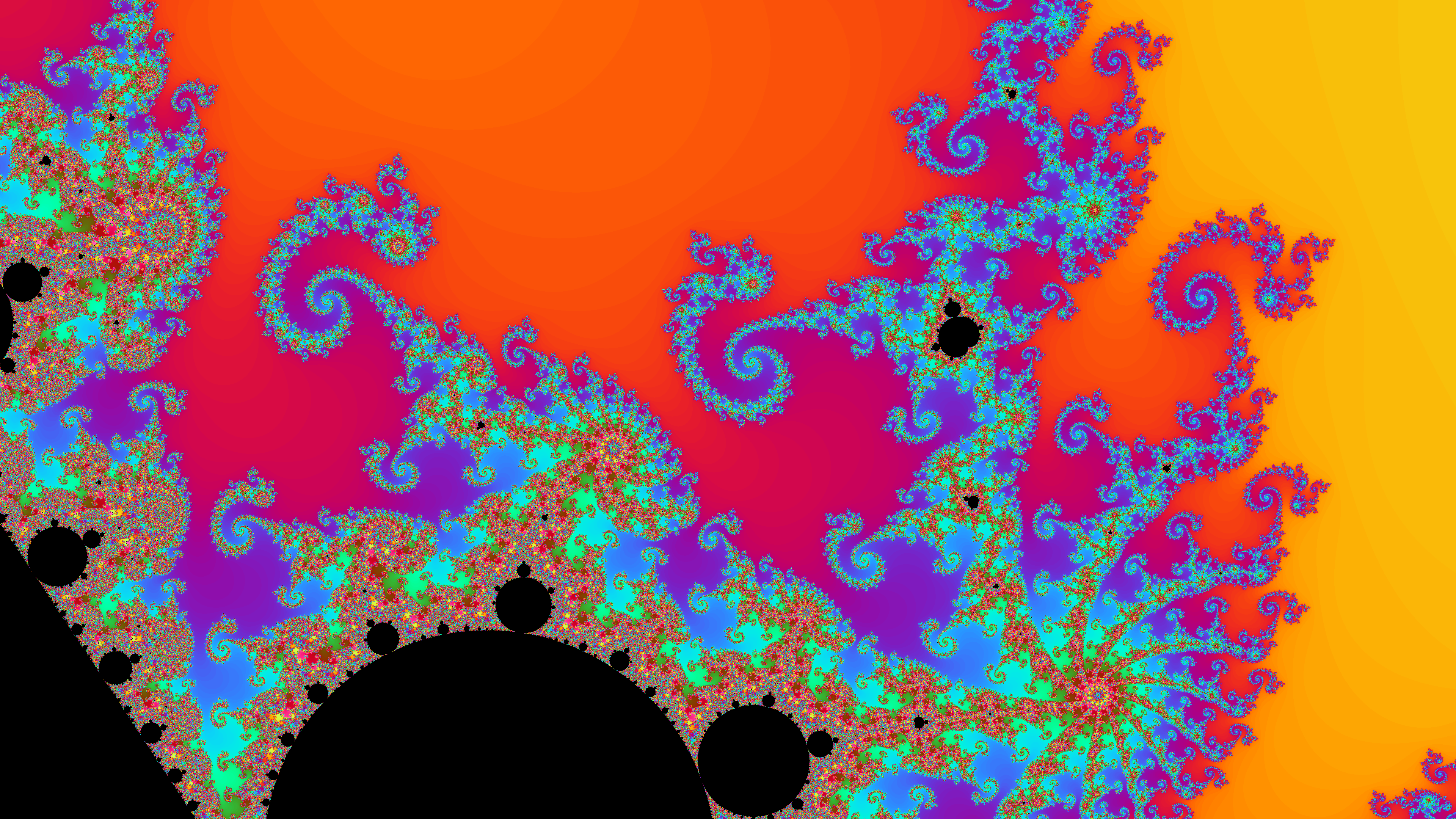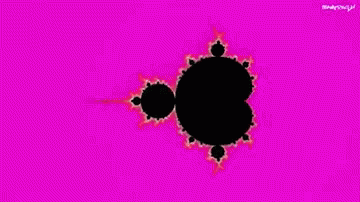# Julia And Mandelbrot Set:

## Explained in brief

1 March 2020 - Ayush GargA quick but detailed explanation of mandelbrot sets, their defining functions and their cool representation

## Complex numbers:

Before starting to talk about the Julia and Mandelbrot fractals, it is necessary to give a brief reference about complex numbers. Basically we can define a complex number as an expression of the form: a + bi

where a and b are real numbers , and i is the square root of -1

Complex numbers are represented on a plane, so that each complex number has an associated point on the plane and vice versa. This identification between points and numbers is what we are interested in knowing in order to understand the representation of the fractals that we deal with in this article. (To learn more about complex numbers see here. )

## The Julia and Mandelbrot Sets

The Julia sets , so called by the mathematician Gaston Julia , are a family of fractal sets that are obtained by studying the behavior of complex numbers when iterated by a function.

A very important family of Julia sets is obtained from simple quadratic functions, such as: F c (z) = z 2 + c , where c is a complex number . The Julia set obtained from this function is denoted J c . The process to obtain this set of Julia de is as follows: Any complex number z is chosen and a sequence of numbers is constructed in the following way:

z 0 = z z 1 = F (z 0 ) = z 0 2 + c z 2 = F (z 1 ) = z 1 2 + c ………………………. z n + 1 = F (z n ) = z n 2 + c If this sequence is bounded, then z is said to belong to the Julia set of parameter c, denoted by Jc; otherwise, if the sequence tends to infinity, z is excluded from it.

It is easy to deduce that obtaining a set of Julia is very laborious, since the previous process would have to be repeated for any complex number z, and deciding in each case whether or not that number belongs to the set Jc.

Due to the infinite number of calculations required to obtain the corresponding graph, it took until the eighties to represent these sets. Thanks to the invention of the computer, they could finally be seen on a screen, even if Mr. Julia never saw them:## The Julia and Mandelbrot Sets

Mandelbrot modifies Julia's iterative process by making point c variable and setting point z 0 = 0. The Mandelbrot set is the set of complex numbers for which the sequence of points obtained by the iterative method

z 0 = 0 z 1 = F (z 0 ) = z 0 2 + c z 2 = F (z 1 ) = z 1 2 + c ………………………. z n + 1 = F (z n ) = z n 2 + c it does not tend to infinity, that is, it is bounded .

If we assign the black color to the points c that give places to bounded sequences and other colors to the other points, depending on how fast they tend to infinity, the representation obtained for the Mandelbrot set is:Let's talk a little about the birth of this complex set.

Mandelbrot had had knowledge of Gaston Julia's work since the mid-1940s, since an uncle of his, a mathematics professor at the Collège de France in Paris, had recommended it to him to continue. At the time, he was not very interested in them, but at the end of the 70s he took them up again, just when he studied in the
application of computer mathematics .

At this time Mandelbrot worked for
IBM at the Thomas B. Watson Research Centerin New York and, at one point, he was commissioned to study the disturbances suffered by electronic transmissions. Mandelbrot discovered that, if a graph were made with the data of said disturbances, self-similarity” could be observed in all the scales of increase.

The most curious thing is that this “self-similarity” could also be seen in other statistical charts such as cotton prices, variations in stock prices, the frequency of English words, the fluctuations of turbulent liquids… The genius of Mandelbrot was to combine Julia's previous work, her studies on disturbances and the use of the computer, speaking for the first time of
"fractality"
of all these phenomena and obtaining the images of the complex that bears his name.

If we zoom in on different parts of the set we can find images like these:### Email

[email protected]